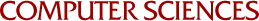Parallel Solution of Extremely Large Knapsack Problems

Michael C Ferris
1989

We shall describe a parallel algorithm for solving the knapsack feasibility problem, also known as the subset sum problem. The use of a random branching technique is described and its implementation on a parallel processor is discussed. Computational results show this to be an effective method for solving large problems. Using this approach we have solved problems with as many as 2 million variables in an average of 800 seconds on the Sequent Symmetry parallel processor. Furthermore, a coarse parallelization overcomes some of the problems that are present when serial algorithms are used to solve the knapsack problem.